# Correlation and scagnostic guided parallel coordinate plots

#### 2020-10-04

PairViz is an R package which provides orderings of objects for visualisation purposes. This vignette demonstrates the use of PairViz constructing parallel coordinate plots, which place emphasis on different data patterns.

## Correlation guided plots of mtcars using Hamiltonians

First, we select six of the variables in `mtcars`, and make a parallel coordinate plot. We will use the variant on a PCP provided by PairViz, which is the function `pcp`.

``````suppressPackageStartupMessages(library(PairViz))
data <- mtcars[,c(1,3:7)]
pcp(data,horizontal=TRUE,lwd=2, col="grey50",
main = "Standard PCP")``````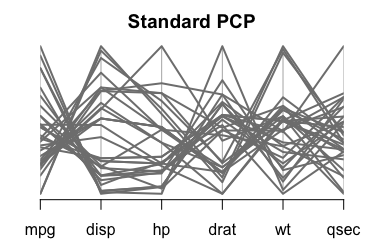The standard PCP will plot the variables in order of appearance in the data frame. It is obvious from this plot for instance that `mpg` and `disp` are negatively correlated. By following the line segments you might be able to see that `mpg` and `hp` are also negatively correlated, but but associations with other variables are difficult to ascertain.

We could use one of the eulerian functions of PairViz to produce orderings of variables where all pairs of variables are adjacent. In the next display, we use `hpaths`, which gives three hamiltonians where each pair of variables is adjacent at least once.

``````o <- hpaths(1:ncol(data),matrix=FALSE)
par(cex.axis=.7)
pcp(data,order=o,horizontal=TRUE,lwd=2, col="grey50",
main = "Hamiltonian decomposition")``````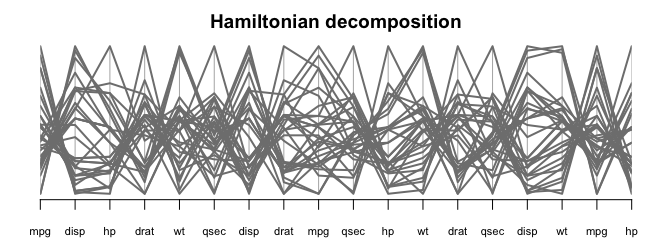From this we see that `mpg` is also strongly negatively correlated with `wt`, `hp` and `drat`, and that there is not a strong association between `mpg` and `drat`.

To make the patterns in the PCP a bit clearer, we will construct an eulerian where high correlation variables appear early on in the sequence.

The code below makes a `dist` with the correlation between variables.

``corw <- as.dist(cor(data))``

As the function `eulerian` constructs a path visiting lower weight edges first (and we want to visit high correlation variables first), we form the path as

``````o <- eulerian(-corw)
o
#>   5 2 3 5 6 1 4 6 2 4 3 6 3 1 2 4 5 1``````

Forming the PCP based on this order we get

``````par(cex.axis=.7)
pcp(data,order=o,horizontal=TRUE,lwd=2, col="grey50",
main = "Weighted eulerian")``````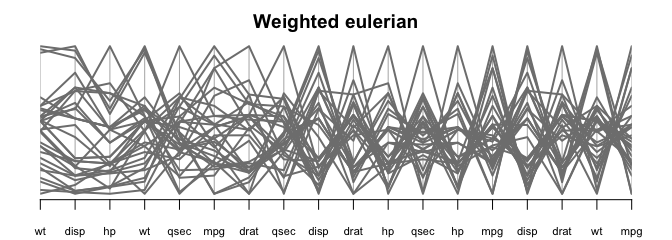The first three panels on the left hand side have many parallel line segments indicative of positively correlated variables. On the right hand side of the PCP, the panels have many high-low line segments which means variables are negatively correlated.

PairViz has an augmented version of a PCP which shows summary measures for each pair of variables, to assist in interpretation.

The code below constructs the summary measures, one column for positive correlations, the second for negative correlations.

``````corw <- dist2edge(corw)
edgew <- cbind(corw*(corw>0), corw*(corw<0))
par(cex.axis=.7)
guided_pcp(data,edgew, path=o,pcp.col="grey50" ,lwd=2,
main="Weighted eulerian with correlation guide",
bar.col = c("lightpink1","lightcyan1"),
bar.ylim=c(-1,1),bar.axes=TRUE)``````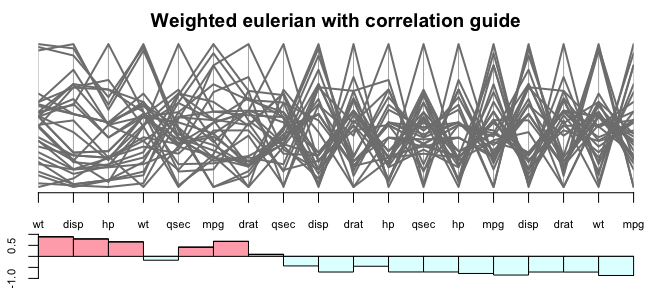The above plot shows clearly that correlation generally decreases from left to right.

If you prefer, omit the guides, but instead colour the panels according to the sign of the correlation.

``````pathw <-  path_weights(corw,o)
corcols <- ifelse(pathw>0, "lightpink1", "lightcyan")
par(cex.axis=.7)
pcp(data,order=o,col="grey50" ,lwd=2,
main="Weighted eulerian with correlation guide",
panel.colors = corcols)``````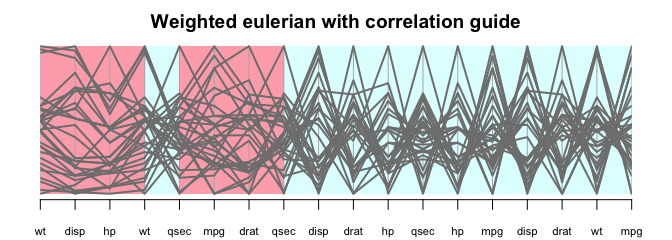The guided_pcp function also has a panel.colors argument, so it is possible to keep the guides and also colour the panels.

## Scagnostic guided plots of sleep data using Hamiltonians

We access the data, remove NAs, transform two highly skewed variables, give variables shortnames, and set up a colour vector for plots.

``````if (!requireNamespace("alr4", quietly = TRUE)){
install.packages("alr4")
}
#> Registered S3 methods overwritten by 'lme4':
#>   method                          from
#>   cooks.distance.influence.merMod car
#>   influence.merMod                car
#>   dfbeta.influence.merMod         car
#>   dfbetas.influence.merMod        car

suppressPackageStartupMessages(library(alr4))
data(sleep1)
data <- na.omit(sleep1)
# these vars changed to factors in alr4, change from alr3
data\$D <- as.numeric(data\$D)
data\$P <- as.numeric(data\$P)
data\$SE <- as.numeric(data\$SE)

# logging the brain and body weights
data[,4:5] <- log(data[,4:5])

# short variable names
colnames(data) <- c("SW","PS" ,"TS" ,"Bd", "Br","L","GP","P" ,"SE" , "D"  )

# colours for cases, split Life values into 3 equal sized groups
cols1 <- scales::alpha(c("red","navy","lightblue3"   ),.6)
cols <- cols1[cut(rank(data\$L),3,labels=FALSE)] ``````

Calculate scagnostics for the data. `sc` is a matrix whose rows are values for 9 scagnostics,

``````library(scagnostics)
library(RColorBrewer)
sc <- scagnostics(data)
scags <- rownames(sc)
scags
#>  "Outlying"  "Skewed"    "Clumpy"    "Sparse"    "Striated"  "Convex"
#>  "Skinny"    "Stringy"   "Monotonic"``````

As we will make plots involving different scagnostics, we will assign colours to scagnostics, for consistency across plots.

``````scag_cols <- rev(brewer.pal(9, "Pastel1"))
names(scag_cols) <- scags``````

Define a utility function which returns a subset of scagnostics, retaining the class attribute.

``````select_scagnostics <- function(sc,names){
sc1 <- sc[names,]
class(sc1) <- class(sc)
return(sc1)
}``````

Consider the outlying scagnostic. Suppose we want to construct a PCP, each variable appearing once, where pairs of variables with a high outlier score appear adjacently in the sequence.

``````scOut <- select_scagnostics(sc,"Outlying")
dOut <- edge2dist(scOut) # dOut is a dist
dOut <- as.matrix(dOut)
rownames(dOut) <- colnames(dOut)<- names(data)``````

`dOut` is a symmetric matrix where each entry gives the outlying score for the scatterplot labelled by the row and column names. Note that the function `edge2dist` relies on the fact that objects of class scagnostics are in order (1,2), (1,3), (2,3),(1,4) etc

To find the variable ordering with the highest (or nearly highest) total outlier score use one of

``````o <- order_best(-dOut, maxexact=10)
o <-order_best(-dOut)
o <-order_tsp(-dOut)``````

The first call to `order_best` above finds the best ordering by a brute force evaluation of all 10! factorial permutations, and so this is a bit slow. Without the `maxexact=10` input, order_best evaluates only a sample of permulations for sequences of length above 9. order_tsp uses a TSP solver from package TSP. We will use the result of the first call to order_best, which gives

``o <-c( 2 , 4 , 1,  5 , 6,  7 , 3 , 8,  9, 10)``

The guided PCP based on this ordering is

``````par(tcl = -.2, cex.axis=.8,mgp=c(3,.3,0))
guided_pcp(data,scOut, path=o,pcp.col=cols,lwd=1.4,
main= "Best Hamiltonian for outliers",bar.col = scag_cols["Outlying"],legend=FALSE,bar.axes=TRUE,bar.ylim=c(0,max(scOut)))``````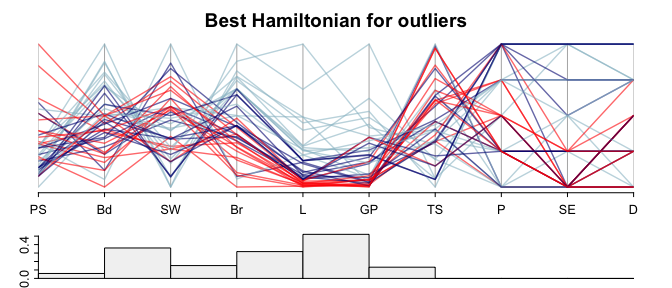Notice that panels involving discrete-valued variables P,SE and D score zero on the outlying index. The L-GP pair of variables has the highest outlier score and two outliers are evident. The outlier cases are the two species (Human and Asian Elephant) with the highest life expectancy (L). Asian Elephant also has the highest value of gestation time (GP)

``````outliers <- order(data\$L, decreasing=T)[1:2]
rownames(data)[outliers]
#>  "Human"          "Asian_elephant"``````

For future use we will construct a colour vector marking these two outliers.

``````colOut <- rep("grey50", nrow(data))
colOut[outliers] <- "red" # Human
colOut[outliers] <- "blue"``````

Suppose next we want to pick the ordering of PCP axes where high scores on the two measures Striated and Sparse are obtained.

``scSS <- t(select_scagnostics(sc,c("Striated", "Sparse")))``

As we did with the calculation for Outliers, we can turn `scSS` into a distance matrix and then use one of `order_best` or `order_tsp` to produces the best ordering.

``````dSS <- edge2dist(scSS[,1])  + edge2dist(scSS[,2])
# You might think edge2dist(scSS[,1]+ scSS[,2]) would work, but as scSS[,1]+ scSS[,2] is
# not of class scagnostics, edge2dist will not fill the dist in the correct order
dSS <- as.matrix(dSS)
rownames(dSS) <- colnames(dSS)<- names(data)
order_best(-dSS,maxexact=10)``````

A shortcut calculation is

``````find_path(-scSS,   order_best,maxexact=10) # for the best path
# or
find_path(-scSS,   order_best) # for a nearly "best" path``````

The best path gives

``o <-  c(4, 10 , 2 , 9 , 1,  7 , 8,  6 , 5 ,3) ``

The guided PCP based on this ordering is

``````par(tcl = -.2, cex.axis=.8,mgp=c(3,.3,0))
guided_pcp(data,scSS, path=o,pcp.col=cols,lwd=1.4,
main= "Best Hamiltonian for Striated + Sparse",
bar.col = scag_cols[c("Striated", "Sparse")],
legend=FALSE,bar.axes=TRUE,bar.ylim=c(0,.6))``````